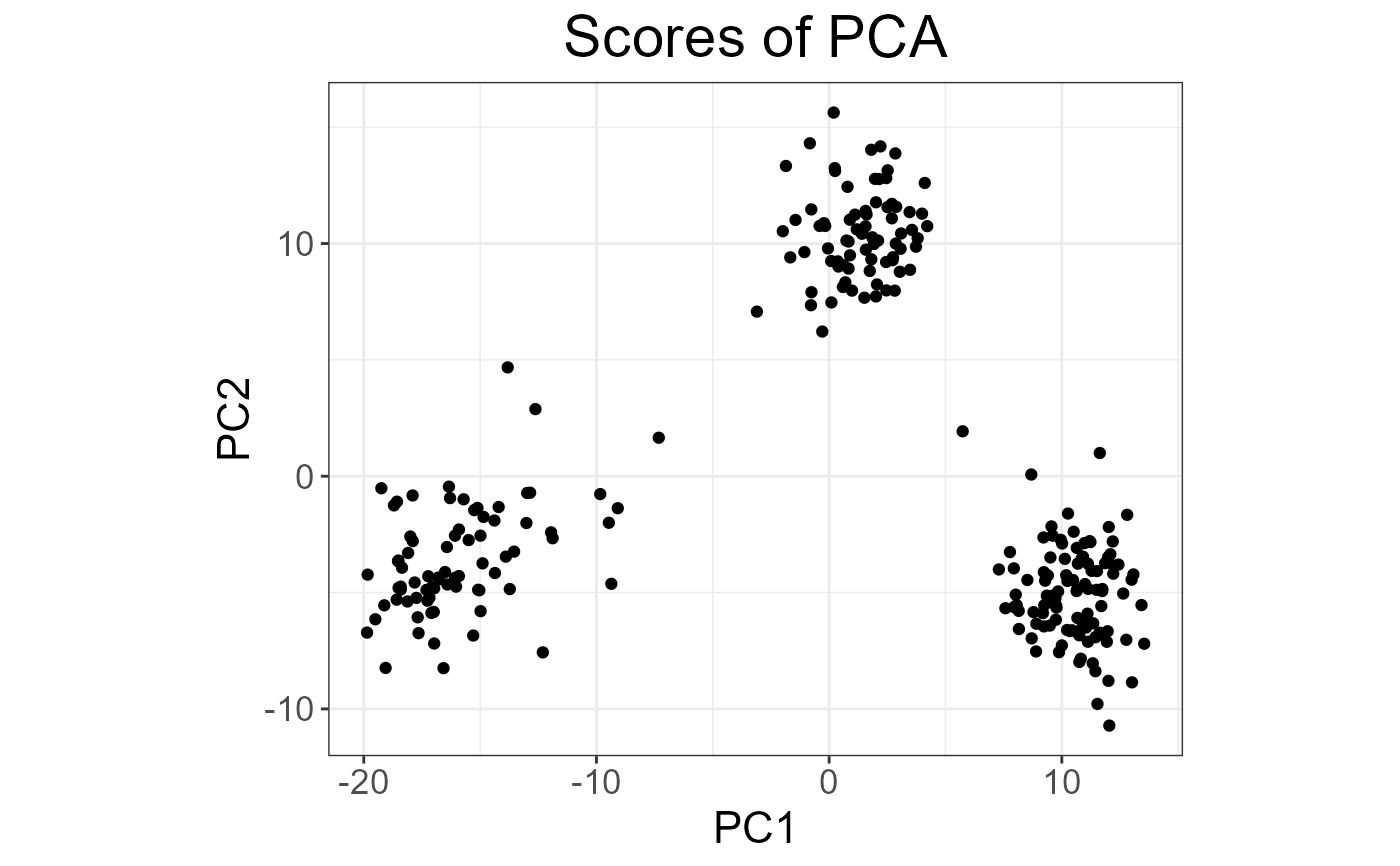An algorithm for partial SVD (or PCA) of a Filebacked Big Matrix based on the algorithm in RSpectra (by Yixuan Qiu and Jiali Mei).
This algorithm is linear in time in all dimensions and is very memory-efficient. Thus, it can be used on very large big.matrices.

big_randomSVD(
X,
fun.scaling = big_scale(center = FALSE, scale = FALSE),
ind.row = rows_along(X),
ind.col = cols_along(X),
k = 10,
tol = 1e-04,
verbose = FALSE,
ncores = 1,
fun.prod = big_prodVec,
fun.cprod = big_cprodVec
)

## Arguments

X

An object of class FBM.

fun.scaling

A function with parameters X, ind.row and ind.col, and that returns a data.frame with $center and $scale for the columns corresponding to ind.col, to scale each of their elements such as followed: $$\frac{X_{i,j} - center_j}{scale_j}.$$ Default doesn't use any scaling. You can also provide your own center and scale by using as_scaling_fun().

ind.row

An optional vector of the row indices that are used. If not specified, all rows are used. Don't use negative indices.

ind.col

An optional vector of the column indices that are used. If not specified, all columns are used. Don't use negative indices.

k

Number of singular vectors/values to compute. Default is 10. This algorithm should be used to compute only a few singular vectors/values.

tol

Precision parameter of svds. Default is 1e-4.

verbose

Should some progress be printed? Default is FALSE.

ncores

Number of cores used. Default doesn't use parallelism. You may use nb_cores.

fun.prod

Function that takes 6 arguments (in this order):

• a matrix-like object X,

• a vector x,

• a vector of row indices ind.row of X,

• a vector of column indices ind.col of X,

• a vector of column centers (corresponding to ind.col),

• a vector of column scales (corresponding to ind.col), and compute the product of X (subsetted and scaled) with x.

fun.cprod

Same as fun.prod, but for the transpose of X.

## Value

A named list (an S3 class "big_SVD") of

• d, the singular values,

• u, the left singular vectors,

• v, the right singular vectors,

• niter, the number of the iteration of the algorithm,

• nops, number of Matrix-Vector multiplications used,

• center, the centering vector,

• scale, the scaling vector.

Note that to obtain the Principal Components, you must use predict on the result. See examples.

## Note

The idea of using this Implicitly Restarted Arnoldi Method algorithm comes from G. Abraham, Y. Qiu, and M. Inouye, FlashPCA2: principal component analysis of biobank-scale genotype datasets, bioRxiv: doi: 10.1101/094714 .
It proved to be faster than our implementation of the "blanczos" algorithm in Rokhlin, V., Szlam, A., & Tygert, M. (2010). A Randomized Algorithm for Principal Component Analysis. SIAM Journal on Matrix Analysis and Applications, 31(3), 1100-1124. doi: 10.1137/080736417 .

## Examples

set.seed(1)

X <- big_attachExtdata()
K <- 10

# Using only half of the data for "training"
n <- nrow(X)
ind <- sort(sample(n, n/2))
test <- big_randomSVD(X, fun.scaling = big_scale(), ind.row = ind, k = K)
str(test)
#> List of 7
#>  $d : num [1:10] 178.5 114.5 91 87.1 86.3 ... #>$ u     : num [1:258, 1:10] -0.1092 -0.0928 -0.0806 -0.0796 -0.1028 ...
#>  $v : num [1:4542, 1:10] 0.00607 0.00739 0.02921 -0.01283 0.01473 ... #>$ niter : num 9
#>  $nops : num 162 #>$ center: num [1:4542] 1.34 1.63 1.51 1.64 1.09 ...
#>  $scale : num [1:4542] 0.665 0.551 0.631 0.55 0.708 ... #> - attr(*, "class")= chr "big_SVD" pca <- prcomp(X[ind, ], center = TRUE, scale. = TRUE) # same scaling all.equal(test$center, pca$center) #>  TRUE all.equal(test$scale,  pca$scale) #>  TRUE # use this function to predict scores class(test) #>  "big_SVD" scores <- predict(test) # scores and loadings are the same or opposite plot(scores, pca$x[, 1:K])plot(test$v, pca$rotation[, 1:K])plot(test\$u)plot(test, type = "scores")# projecting on new data
ind2 <- setdiff(rows_along(X), ind)
scores.test2 <- predict(test, X, ind.row = ind2)
scores.test3 <- predict(pca, X[-ind, ])
plot(scores.test2, scores.test3[, 1:K])